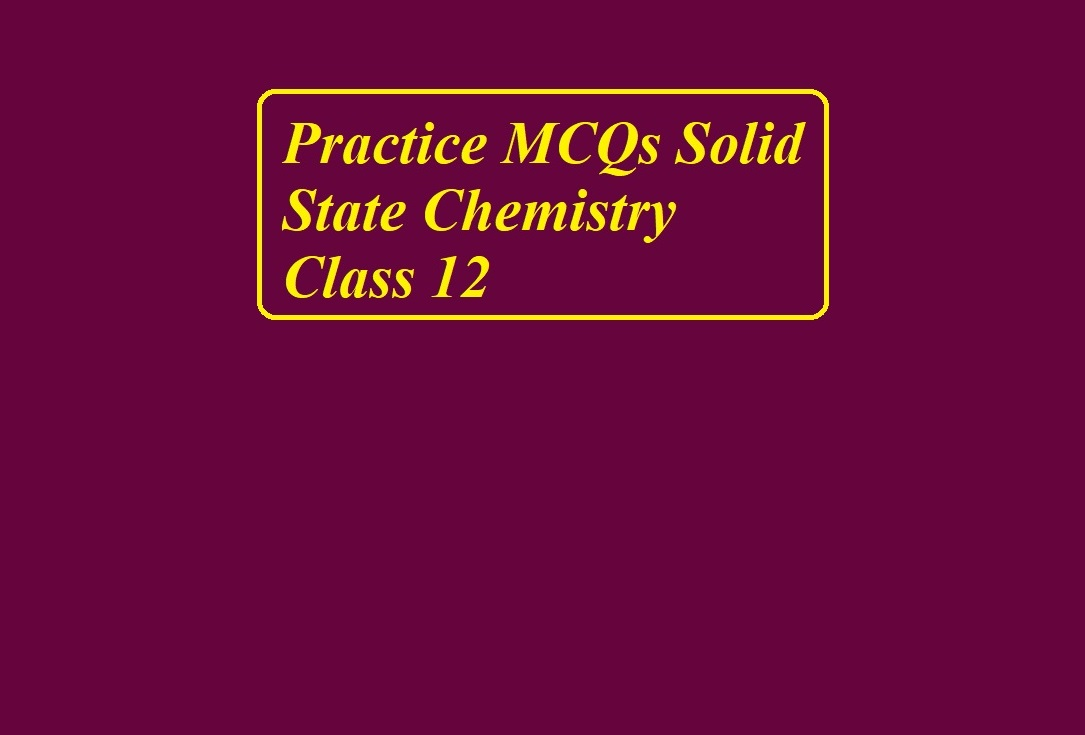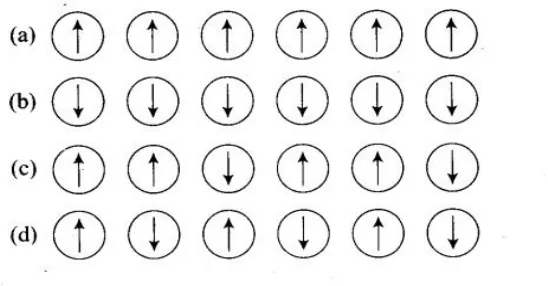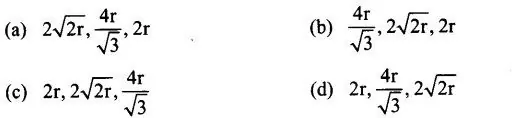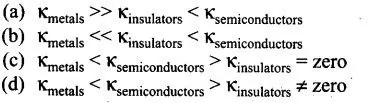# Practice MCQs Solid State Chemistry Class 12# Practice MCQs Solid State Chemistry Class 12

Practice MCQs Solid State Chemistry Class 12

Que 1. Which of the following arrangement shows the schematic alignment of magnetic moments of antiferromagnetic substances?Que 2. Which of the following is true about the value of the refractive index of quartz glass?
(a) Same in all directions                            (b) Different in different directions
(c) Cannot be measured                              (d) Always zero

Que 3. Which of the following statement is not true about amorphous solids?
(a) On heating they may become crystalline at a certain temperature
(b) They may become crystalline on keeping for a long time
(c) Amorphous solids can be molded by heating
(d) They are anisotropic in nature

Que 4. The sharp melting point of crystalline solids is due to
(a) a regular arrangement of constituent particles observed over a short distance in the crystal lattice
(b) a regular arrangement of constituent particles observed over a long distance in the crystal lattice
(c) the same arrangement of constituent particles in different directions
(d) different arrangement of constituent particles in different directions

Que 5. Iodine molecules are held in the crystals lattice by
(a) London forces                                           (b) dipole-dipole interactions
(c) covalent bonds                                          (d) coulombic forces

Que 6. Which of the following is a network solid?
(a) SO2 (solid)                  (b) I2                     (c) Diamond                     (d) H2O (ice)

Que 7. Which of the following is not the characteristic of ionic solids?
(a) Very low value of electrical conductivity in the molten state
(b) Brittle nature
(c) Very strong forces of interactions
(d) Anisotropic nature

Practice MCQs Solid State Chemistry Class 12

Que 8. Graphite is a good conductor of electricity due to the presence of
(a) lone pair of electrons                                           (b) free valence electrons
(c) cations                                                                  (d) anions

Que 9. Which of the following oxide behaves as a conductor or insulator depending upon temperature?
(a) TiO                            (b) Si02                       (c) TiO3                    (d) MgO

Que 10. Which of the following oxide shows electrical properties like metals?
(a) SO2                           (b) MgO                     (c)SO2(s)                    (d) CrO2

Que 11. The lattice site in a pure crystal cannot be occupied by
(a) molecule                    (b) ion                       (c) electron                  (d) atom

Que 12. Graphite cannot be classified as
(a) conducting solid                                                      (b) network solid
(c) covalent solid                                                          (d) ionic solid

Que 13. Cations are present in the interstitial sites in
(a) Frenkel defect                                                         (b) Schottky defect
(c) vacancy defect                                                        (d) metal deficiency defect

Que 14. The Schottky defect is observed in crystals when
(a) some cations move from their lattice site to interstitial sites
(b) an equal number of cations and anions are missing from the lattice
(c) some lattice sites are occupied by electrons
(d) some impurity is present in the lattice

Practice MCQs Solid State Chemistry Class 12

Que 15. Which of the following is true about the charge acquired by p-type semiconductors?
(a) Positive         (b) Neutral      (c) Negative    (d) Depends on concentration of p impurity

Que 16. To get an n-type semiconductor from silicon, it should be doped with a substance with valency
(a) 2                   (b) 1                (c) 3                (d) 5

Que 17. The total number of tetrahedral voids in the face-centered unit cell is
(a) 6                   (c) 10              (c) 8                (d) 12

Que 18. Which of the following point defects are shown by AgBr (s) crystals?
(A) Schottky defect                                    (B) Frenkel defect
(C) Metal excess defect                              (D) Metal deficiency defect
(a) A and B                                                 (b) C and D
(c) A and C                                                 (d) B and D

Que 19. In which pair most efficient packing is present?
(a) hcp and bcc                                                (b) hcp and ccp
(c) bcc and ccp                                                (d) bcc and simple cubic cell

Que 20. The percentage of empty space in a body-centered cubic arrangement is
(a) 74                             (b) 68                         (c) 32              (d) 26

Que 21. Which of the following statement is not true about the hexagonal close packing?
(a) The coordination number is 12
(b) It has 74% packing efficiency
(c) Tetrahedral voids of the second layer are covered by the spheres of the third layer
(d) In this arrangement, spheres of the fourth layer are exactly aligned with those of the first layer.

Practice MCQs Solid State Chemistry Class 12

Que 22. In which of the following structure coordination number for cations and anions in the packed structure will be the same?
(a) Cl ions form fee lattice and Na+ ions occupy all octahedral voids of the unit cell.
(b) Ca2+ ions form fee lattice and F ions occupy all the eight tetrahedral voids of the unit cell
(c) O2- ions form fee lattice and Na+ ions occupy all the eight tetrahedral voids of the unit cell
(d) S2- ions form fee lattice and Zn2+ ions go into alternate tetrahedral voids of the unit cell.

Que 23. What is the coordination number in a square close-packed structure in two dimensions?
(a) 2                               (b) 3                            (c) 4                            (d) 6

Que 24. Which kind of defect is introduced by doping?
(a) Dislocation defect                                       (b) Schottky defect
(c) Frenkel defect                                             (d) Electronic defect

Que 25. Silicon doped with electron-rich impurity forms
(a) p-type semiconductor                                  (b) n-type semiconductor
(c) intrinsic semiconductor                               (d) insulator

Que 26. Which of the following statement is not true?
(a) Paramagnetic substances are weakly attracted by the magnetic field
(b) Ferromagnetic substances cannot be magnetized permanently
(c) The domains in antiferromagnetic substances are oppositely oriented with respect to each other
(d) Pairing of electrons cancels their magnetic moment in the diamagnetic substances.

Que 27. Which of the following is not true about the ionic solids?
(a) Bigger ions form the close-packed structure
(b) Smaller ions occupy either the tetrahedral or the octahedral voids depending upon their size
(c) Occupation of all the voids is not necessary
(d) The fraction of octahedral or tetrahedral voids occupied depends upon the radii of the ions occupying the voids.

Que 28. A ferromagnetic substance becomes a permanent magnet when it is placed in a magnetic field because
(a) all the domains get oriented in the direction of the magnetic field
(b) all the domains get oriented ill the direction opposite to the direction of the magnetic field
(c) domains get oriented randomly
(d) domains are not affected by the magnetic field.

Practice MCQs Solid State Chemistry Class 12

Que 29. The correct order of the packing efficiency in different types of unit cells is…………
(a) fee < bee < simple cubic                      (b) fee > bee simple cubic
(c) fee < bee > simple cubic                      (d) bee < fee > simple cubic

Que 30. Which of the following defects is also known as dislocation defect?
(a) Frenkel defect                                       (b) Schottky defect
(c) Non-stoichiometric defect                    (d) Simple interstitial defect

Que 31. In the cubic close packing, the unit cell has
(a) 4 tetrahedral voids each of which is shared by four adjacent unit cells
(b) 4 tetrahedral voids within the unit cell
(c) 8 tetrahedral voids each of which is shared by four adjacent unit cells
(d) 8 tetrahedral voids within the unit cells.

Que 32. The edge lengths of the unit cells in terms of the radius of spheres constituting fee, bcc, and simple cubic unit cells are respectivelyQue 33. Which of the following defects decreases the density?
(a) Interstitial defect                                      (b) Vacancy defect
(c) Frenkel defect                                          (d) Schottky defect

Que 34. Which of the following is not true about voids formed in three-dimensional hexagonal close-packed structures?
(a) A tetrahedral void is formed when a sphere of the second layer is present above the triangular void in the first layer
(b) All the triangular voids are not covered by the spheres of the second layer
(c) Tetrahedral voids are formed when the triangular voids in the second layer lie above the triangular voids in the first layer and the triangular voids in the first layer and the triangular shapes of these voids do not overlap
(d) Octahedral voids are formed when the triangular voids in the second layer exactly overlap with similar voids in the first layer.

Que 35. The value of the magnetic moment is zero in the case of antiferromagnetic substances because of the domains …
(a) get oriented in the direction of the applied magnetic field
(b) get oriented opposite to the direction of the applied magnetic field
(c) are oppositely oriented with respect to each other without the application of magnetic field
(d) cancel out each other’s magnetic moment

Practice MCQs Solid State Chemistry Class 12

Que 36. Which of the following statements are not true?
(a) Vacancy defect results in a decrease in the density of the substance
(b) Interstitial defects result in an increase in the density of the substance
(c) Impurity defect has no effect on the density of the substance
(d) Frenkel defect results in an increase in the density of the substance

Que 37. Which of the following statements are true about metals?
(a) Valence band overlap with the conduction band
(b) The gap between the valence band and conduction band is negligible
(c) The gap between the valence band and conduction band cannot be determined
(d) Valence band may remain partially filled.

Que 38. Under the influence of the electric field, which of the following statements are true about the movement of electrons and holes in a p-type semiconductor?
(a) Electron will move towards the positively charged plate through electron holes
(b) Holes will appear to be moving towards the negatively charged plate
(c) Both electrons and holes appear to move towards the positively charged plate
(d) Movement of electrons is not related to the movement of holes

Que 39. Which of the following statements are true about semiconductors?
(a) Silicon doped with an electron-rich impurity is a p-type semiconductor
(b) Silicon doped with an electron-rich impurity is an n-type semiconductor
(c) Delocalized electrons increase the conductivity of doped silicon
(d) An electron vacancy increases the conductivity of an n-type semiconductor.

Que 40. An excess of potassium ions makes KCl crystals appear violet or Lilac in colour since
(a) some of the anionic sites are occupied by an unpaired electron
(b) some of the anionic sites are occupied by a pair of electrons
(c) there are vacancies at some anionic sites
(d) F-centers are created which impart colour to the crystals.

Practice MCQs Solid State Chemistry Class 12